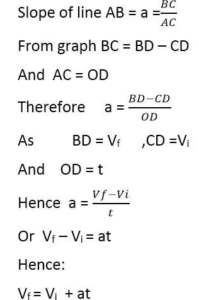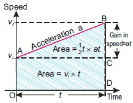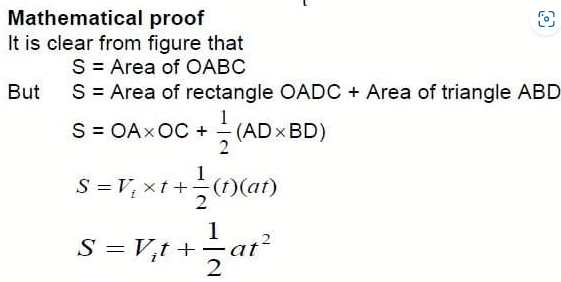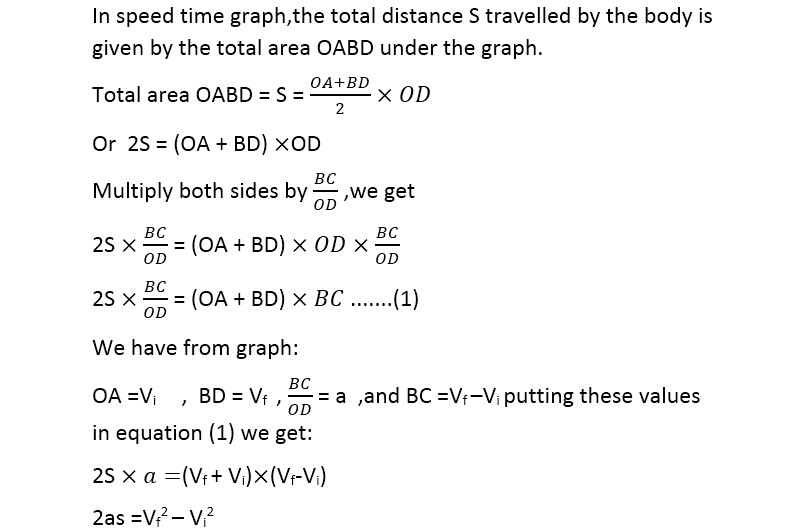Mechanics

# Kinematic Equations in Physics List

Kinematics is the branch of mechanics dealing with the motion of bodies without reference to mass or force. There are three kinematic equations in Physics for bodies moving with uniform acceleration. These equations relate initial velocity, final velocity, acceleration, time, and distance covered by a moving body.
For equations of motion derivation, we assume that the motion is along a straight line. Hence, we consider only the magnitude of displacements, velocities, and acceleration.

## The first equation of motion derivation by graphical method

Consider a body moving with initial velocity Vi in a straight line with uniform acceleration a. Its velocity becomes Vf after time t. The motion of the body is described by the speed-time graph represented by line AB. The slope of line AB is acceleration a. The total distance covered by the body is shown by the shaded area under the line AB. Kinematic equations of motion can be obtained easily from this graph.

Speed time graph for the motion of a body is shown in the figure. The slope of line AB gives the acceleration of a body.## The second equation of motion derivation by graphical method

Consider a body is moving with initial velocity ‘Vi’ in a straight line with uniform acceleration ‘a’.Lets its velocity becomes ‘Vf’ after time t. The motion of the body is described by the speed-time graph by line AB as shown in the figure below. The total distance ‘S’ is covered by the body is equal to the total area OABD under the graph.It is known as the 2nd equation of motion.## Third (3rd) equation of motion by graphical method

Consider a body moving with initial velocity ‘vi’ in a straight line with uniform acceleration ‘a’.Let its velocity becomes Vf after time ‘t’.The motion of the body is described by the speed-time graph as shown in the figure by line AB. The total distance ‘S’traveled by the body is given by the total area OABD under the graph.It is 3rd equation of motion.
The conditions under which these equations can be applied:
1: Motion should be 1-dimensional.
2: Acceleration should be uniform.
3: Frame of reference should be inertial.
Related topics: